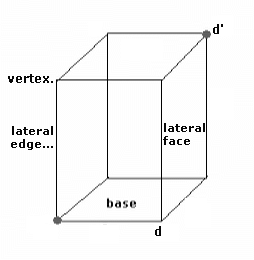### Prism

The Prism is a three-dimensional solid figure formed by two parallel polygons and congruent called bases. The number of sides of one of the bases gives the name of a prism, such as triangular, quadrangular, pentagonal and octagonal for 3, 4, 5 and 8 sides,respectively.
The Identical ends are called, prism bases. The shapes of the ends give the name to the prism, such as triangular, quadrangular, pentagonal and octagonal.
Read full text in Prism.
Enter data using the point as a decimal separator. Ex 1,250.37 enter: 1250.37 , the results will be shown after a click on CalculatePrism Decimal places: Base side: Number of sides: Height: Total surface: Lateral surface: Area of the bases: Volume: Height: Lateral diagonal: Base incircle radius: Base excircle radius: Note:

Note:The accuracy of the calculator and its applicability to particular cases is not guaranteed.